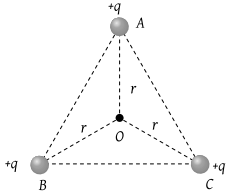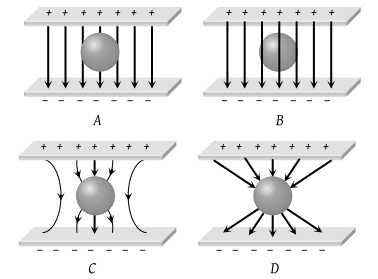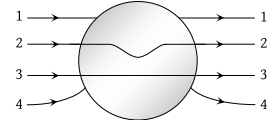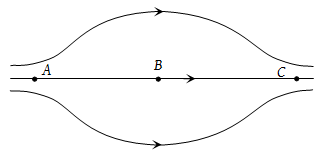ABC is an equilateral triangle. Charges +q are placed at each corner. The electric intensity at O will be(1) $\frac{1}{4\pi {\epsilon }_{0}}\frac{q}{{r}^{2}}$

(2) $\frac{1}{4\pi {\epsilon }_{0}}\frac{q}{r}$

(3) Zero

(4) $\frac{1}{4\pi {\epsilon }_{0}}\frac{3q}{{r}^{2}}$

Concept Questions :-

Electric field
High Yielding Test Series + Question Bank - NEET 2020

Difficulty Level:

The magnitude of electric field intensity E is such that, an electron placed in it would experience an electrical force equal to its weight is given by

(1) mge

(2) $\frac{mg}{e}$

(3) $\frac{e}{mg}$

(4) $\frac{{e}^{2}}{{m}^{2}}g$

Concept Questions :-

Electric field
High Yielding Test Series + Question Bank - NEET 2020

Difficulty Level:

A charge particle is free to move in an electric field. It will travel

(1) Always along a line of force

(2) Along a line of force, if its initial velocity is zero

(3) Along a line of force, if it has some initial velocity in the direction of an acute angle with the line of force

(4) None of the above

Concept Questions :-

Electric field
High Yielding Test Series + Question Bank - NEET 2020

Difficulty Level:

An uncharged sphere of metal is placed in between two charged plates as shown. The lines of force look like(1) A

(2) B

(3) C

(4) D

Concept Questions :-

Electric field
High Yielding Test Series + Question Bank - NEET 2020

Difficulty Level:

The electric field near a conducting surface having a uniform surface charge density σ is given by

(1) $\frac{\sigma }{{\epsilon }_{0}}$ and is parallel to the surface

(2) $\frac{2\sigma }{{\epsilon }_{0}}$ and is parallel to the surface

(3) $\frac{\sigma }{{\epsilon }_{0}}$ and is normal to the surface

(4) $\frac{2\sigma }{{\epsilon }_{0}}$ and is normal to the surface

Concept Questions :-

Electric field
High Yielding Test Series + Question Bank - NEET 2020

Difficulty Level:

The magnitude of electric field E in the annular region of a charged cylindrical capacitor

(1) Is same throughout

(2) Is higher near the outer cylinder than near the inner cylinder

(3) Varies as 1/r, where r is the distance from the axis

(4) Varies as 1/r2, where r is the distance from the axis

Concept Questions :-

Electric field
High Yielding Test Series + Question Bank - NEET 2020

Difficulty Level:

A metallic solid sphere is placed in a uniform electric field. The lines of force follow the path(s) shown in figure as(1) 1

(2) 2

(3) 3

(4) 4

Concept Questions :-

Electric field
High Yielding Test Series + Question Bank - NEET 2020

Difficulty Level:

The figure shows some of the electric field lines corresponding to an electric field. The figure suggests(1) EA > EB > EC

(2) EA = EB = EC

(3) EA = EC > EB

(4) EA = EC < EB

Concept Questions :-

Electric field
High Yielding Test Series + Question Bank - NEET 2020

Difficulty Level:

A hollow insulated conducting sphere is given a positive charge of 10μC. What will be the electric field at the centre of the sphere if its radius is 2 meters

(1) Zero

(2) 5μ Cm–2

(3) 20μ Cm–2

(4) 8μ Cm–2

Concept Questions :-

Electric field
High Yielding Test Series + Question Bank - NEET 2020

Difficulty Level:

An electron of mass me initially at rest moves through a certain distance in a uniform electric field in time t1. A proton of mass mp also initially at rest takes time t2 to move through an equal distance in this uniform electric field. Neglecting the effect of gravity, the ratio of t2/ t1 is nearly equal to

(1) 1

(2) ${\left({m}_{p}/{m}_{e}\right)}^{1/2}$

(3) ${\left({m}_{e}/{m}_{p}\right)}^{1/2}$

(4) 1836

Concept Questions :-

Electric field